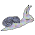### Circle packing in R (again)

Back in 2010 I posted some R code for circle packing. Now, just five years later, I've ported the code to Rcpp and created a little package which you can find at GitHub.

The main function is `circleLayout` which takes a set of overlapping circles and tries to find a non-overlapping arrangement for them. Here's an example:

And here's the code:
``````
# Create some random circles, positioned within the central portion
# of a bounding square, with smaller circles being more common than
# larger ones.

ncircles <- 200
limits <- c(-50, 50)
inset <- diff(limits) / 3
rmax <- 20

xyr <- data.frame(
x = runif(ncircles, min(limits) + inset, max(limits) - inset),
y = runif(ncircles, min(limits) + inset, max(limits) - inset),
r = rbeta(ncircles, 1, 10) * rmax)

# Next, we use the `circleLayout` function to try to find a non-overlapping
# arrangement, allowing the circles to occupy any part of the bounding square.
# The returned value is a list with elements for the layout and the number
# of iterations performed.

library(packcircles)

res <- circleLayout(xyr, limits, limits, maxiter = 1000)
cat(res\$niter, "iterations performed")

# Now draw the before and after layouts with ggplot

library(ggplot2)
library(gridExtra)

## plot data for the `before` layout
dat.before <- circlePlotData(xyr)

## plot dta for the `after` layout returned by circleLayout
dat.after <- circlePlotData(res\$layout)

doPlot <- function(dat, title)
ggplot(dat) +
geom_polygon(aes(x, y, group=id), colour="brown", fill="burlywood", alpha=0.3) +
coord_equal(xlim=limits, ylim=limits) +
theme_bw() +
theme(axis.text=element_blank(),
axis.ticks=element_blank(),
axis.title=element_blank()) +
labs(title=title)

grid.arrange(
doPlot(dat.before, "before"),
doPlot(dat.after, "after"),
nrow=1)

``````

1.Wow! It's magic. Thank you for posting this.

This is an interesting problem to solve in code. Very cool.

1.You're welcome Phillip. If you try it out let me know it goes.

2.I'm having issues compiling the package:

* installing *source* package 'packcircles' ...
** libs
Warning: running command 'make -f "C:/PROGRA~1/R/R-31~1.3/etc/x64/Makeconf" -f "C:/PROGRA~1/R/R-31~1.3/share/make/winshlib.mk" SHLIB_LDFLAGS='\$(SHLIB_CXXLDFLAGS)' SHLIB_LD='\$(SHLIB_CXXLD)' SHLIB="packcircles.dll" WIN=64 TCLBIN=64 OBJECTS="RcppExports.o packcircles.o"' had status 127
ERROR: compilation failed for package 'packcircles'

1.Hi Jim,
Was that the entire error trace ? I'm building on a windows 7 box with Rtools 3.1.0.1942 and devtools 1.7.0.

In case I'd missed including something essential in the repo, I just tried cloning it into a new folder, opening the project in RStudio and building it from there. There were no errors.

If you can't get it to work I could send you a zip file of the built package to install.

2.I also just tried doing `devtools::install_github("mbedward/packcircles")` which also worked. For the moment I'm guessing the problem has to do with your local setup.

### Fitting an ellipse to point data

Some time ago I wrote an R function to fit an ellipse to point data, using an algorithm developed by Radim Halíř and Jan Flusser 1 in Matlab, and posted it to the r-help list . The implementation was a bit hacky, returning odd results for some data. A couple of days ago, an email arrived from John Minter asking for a pointer to the original code. I replied with a link and mentioned that I'd be interested to know if John made any improvements to the code. About ten minutes later, John emailed again with a much improved version ! Not only is it more reliable, but also more efficient. So with many thanks to John, here is the improved code: fit.ellipse <- function (x, y = NULL) { # from: # http://r.789695.n4.nabble.com/Fitting-a-half-ellipse-curve-tp2719037p2720560.html # # Least squares fitting of an ellipse to point data # using the algorithm described in: # Radim Halir & Jan Flusser. 1998. # Numerically stable direct least squares fitting of ellipses

### packcircles version 0.2.0 released

Version 0.2.0 of the packcircles package has just been published on CRAN. This package provides functions to find non-overlapping arrangements of circles in bounded and unbounded areas. The package how has a new circleProgressiveLayout function. It uses an efficient deterministic algorithm to arrange circles by consecutively placing each one externally tangent to two previously placed circles while avoiding overlaps. It was adapted from a version written in C by Peter Menzel who lent his support to creating this R/Rcpp version. The underlying algorithm is described in the paper: Visualization of large hierarchical data by circle packing by Weixin Wang, Hui Wang, Guozhong Dai, and Hongan Wang. Published in Proceedings of the SIGCHI Conference on Human Factors in Computing Systems, 2006, pp. 517-520 (Available from ACM ). Here is a small example of the new function, taken from the package vignette: library(packcircles) library(ggplot2) t <- theme_bw() + theme(panel.grid =

### Graph-based circle packing

The previous two posts showed examples of a simple circle packing algorithm using the packcircles package (available from CRAN and GitHub ). The algorithm involved iterative pair-repulsion to jiggle the circles until (hopefully) a non-overlapping arrangement emerged. In this post we'll look an alternative approach. An algorithm to find an arrangement of circles satisfying a prior specification of circle sizes and tangencies was described by Collins and Stephenson in their 2003 paper in Computation Geometry Theory and Applications. A version of their algorithm was implemented in Python by David Eppstein as part of his PADS library (see CirclePack.py ). I've now ported David's version to R/Rcpp and included it in the packcircles package. In the figure below, the graph on the left represents the desired pattern of circle tangencies: e.g. circle 7 should touch all of, and only, circles 1, 2, 6 and 8. Circles 5, 7, 8 and 9 are internal , while the remaining circles are exter